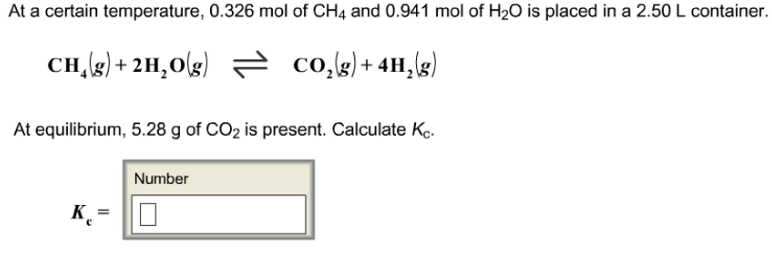# Problem: At a certain temperature, 0.326 m of CH 4 and 0.941 mol of H2O is placed in a 2.50 L container. CH4(g) + 2H2O(g) ⇌ CO2(g) + 4H2(g) At equilibrium, 5.28 g of CO2 is present. Calculate Kc.

###### FREE Expert Solution
91% (157 ratings)###### Problem Details

At a certain temperature, 0.326 m of CH 4 and 0.941 mol of H2O is placed in a 2.50 L container.

CH4(g) + 2H2O(g) ⇌ CO2(g) + 4H2(g)

At equilibrium, 5.28 g of CO2 is present. Calculate Kc